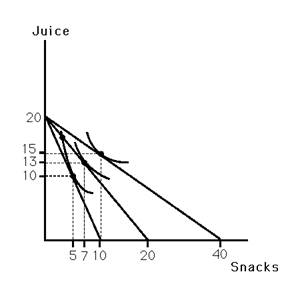### CFA Practice Question

There are 923 practice questions for this topic.

### CFA Practice Question

This figure shows Bobby's indifference map for juice and snacks. Also shown are three budget lines resulting from different prices for snacks assuming he has \$20 to spend on these goods. Which of the following points are on Bobby's demand curve for snacks?A. p = 2, q = 10
B. p = 2, q = 5
C. p = 1, q = 20

When their price is \$2, he can buy 10 snacks. However, buying 5 snacks and 10 bottles of juice will yield a higher indifference curve.

User Comment
johntan1979 Seriously don't understand the answer. If budget is \$20, then it can only be A or C where pq = \$20
sapu He has to buy both goods, so if he buys all snacks( \$20) he will left with nothing to buy juices.In A and C all is spent on snacks..Only in b option combinations are possible.
gill15 All the points the graph above where the indifference curve intersects the budgent constraint curve are on the Demand Curve for Snacks(we're just changing the price of snack). The points P =0.5 , Q=10 from the intersection to the far right is also included as is the point P = 1 and Q=7 from the middle interection.
Seancfa1 ?
Shaan23 This question is not difficult.
1. We're looking for the highest indifference curve
2. At P=2 - we know we're ONLY looking at the steepest budget constrain curve(First One).
- On that curve at Q=10 the indifference curve would be way at the bottom
- On that curve at Q=5 the indifference curve is much higher.
3. Check the last case which is P=1, which would be the budget constraint curve in the middle.
- at Q = 20 the indifference curve is way at the bottom again.

so we know its B
schweitzdm Shaan23, how do you know which curve to examine given the P value?
kay136 Another way to explain:

A) price * quantity (2*10)= \$20 to spend on snacks. no money to spend on juices- can't be right.

B) p*q= \$10. At a quantity of 5 snacks Bobby has left over money to buy the 10 juices.

c) 1*20 = \$20 again bobby has no money to buy juices if he buys 20 snacks at \$1 each
Freddie33 Bobby is yet another contribution to the global obesity crisis